Function Repository Resource:

# PedalCurve

Compute the pedal of a curve

Contributed by: Alfred Gray
 ResourceFunction["PedalCurve"][c,t] computes the pedal curve of a curve c with parameter t. ResourceFunction["PedalCurve"][c,p,t] computes the pedal curve from the point p with parameter t.

## Details and Options

The curve c must be defined in two dimensions.
If no point p is given, the origin {0,0} is used.
The pedal of a given curve c from a fixed point p is the locus of the foot of the perpendicular from p to the tangent to c.

## Examples

### Basic Examples (3)

Define a cardioid:

 In:=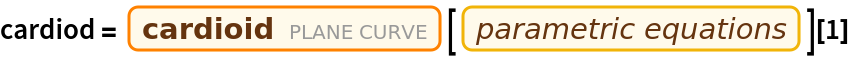Out=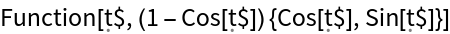Compute the pedal curve of the cardioid:

 In:=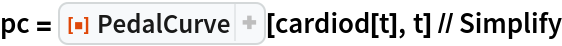Out=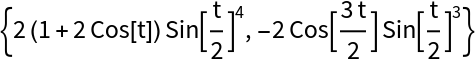Plot the cardioid (blue) along with its pedal curve (orange):

 In:=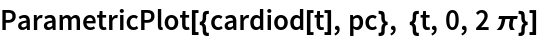Out=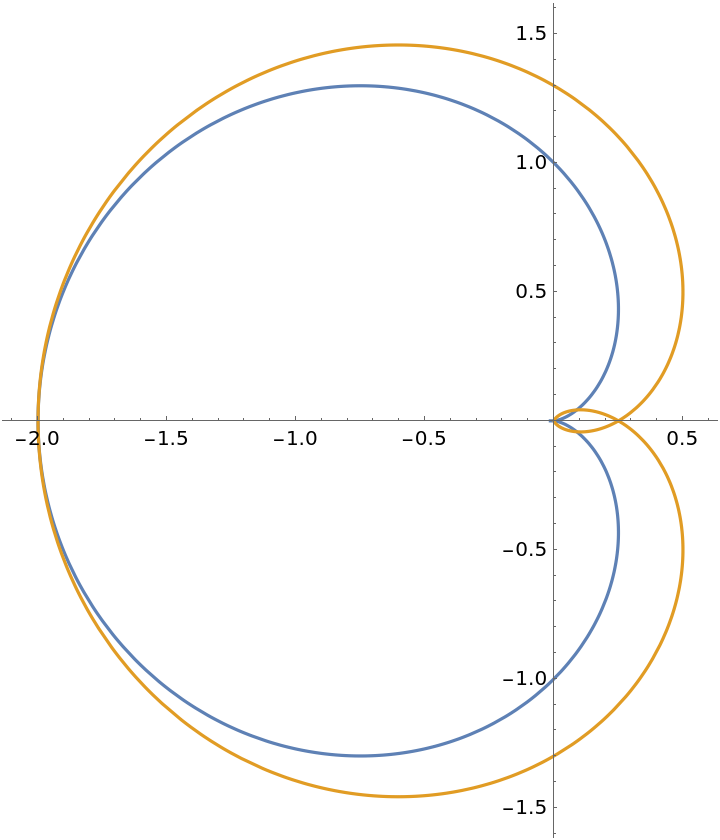### Scope (2)

The pedal curve of an ellipse from different points:

 In:=Out=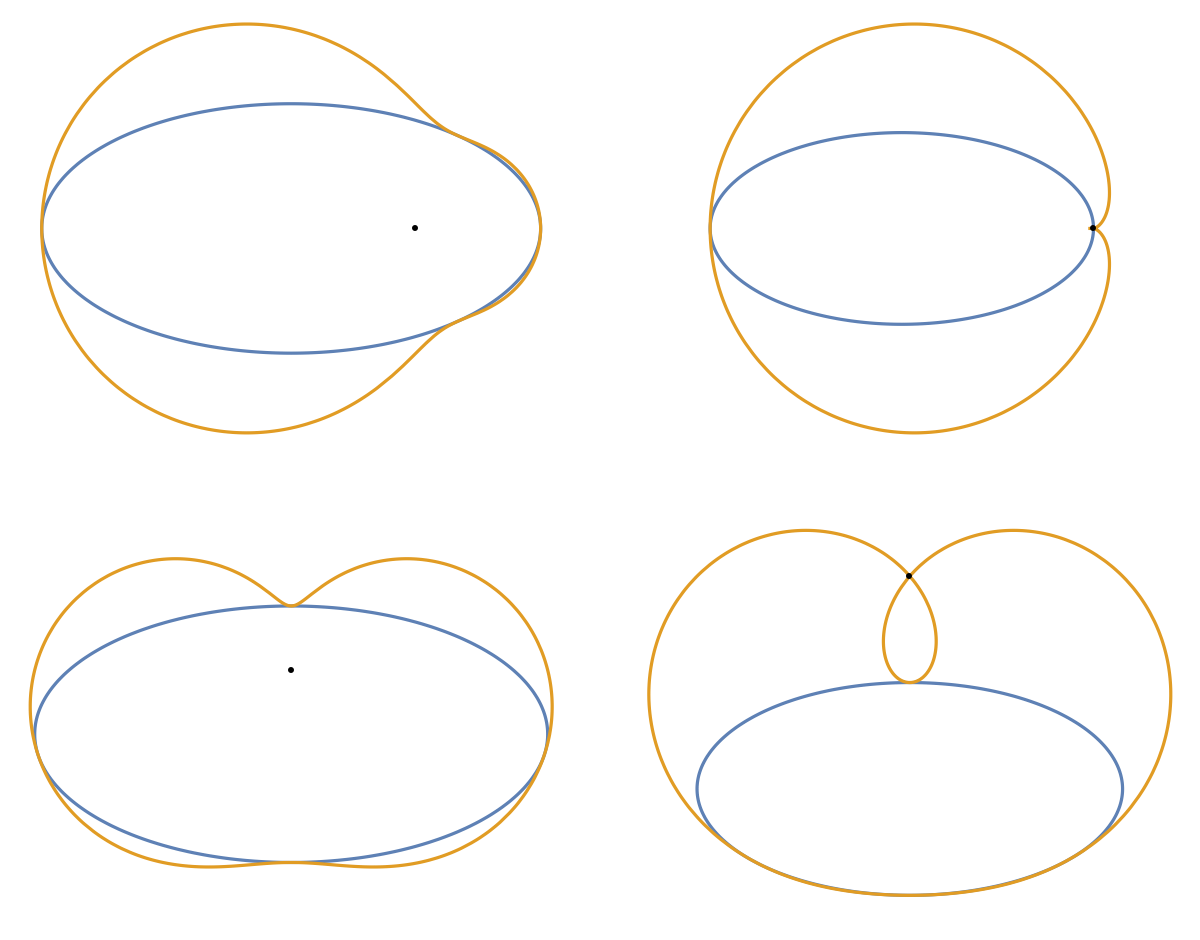Nested pedal curves:

 In:=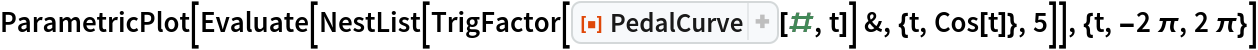Out=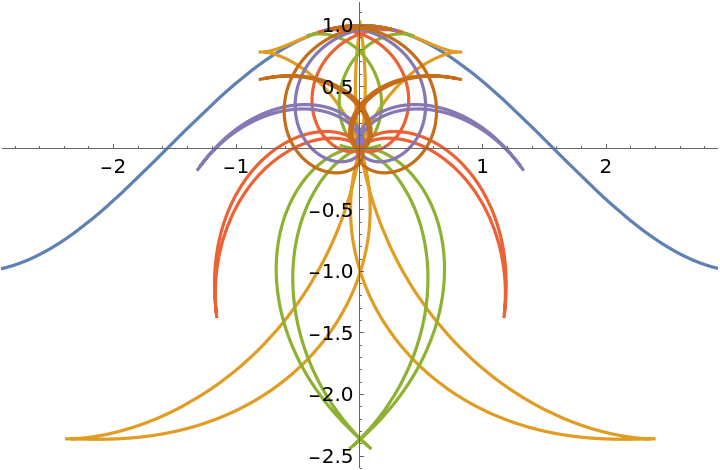Enrique Zeleny

## Version History

• 1.0.0 – 20 February 2020